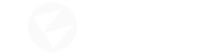Hello, I want to add sun glittering effect to water shader as in the picture.

I know with roughness and metalness the engine does this but I want to make my own effect. I tried the methods I found on the internet but no success. For example this code:

``````float3 normal = normalize(In.Normal.xyz);
float3 v = normalize(mul(In.Pos,matWorld) - vecViewPos.xyz/vecViewPos.w);
Out.Tex2 = reflect(v,normal);
float sunlight = pow(saturate(dot(Out.Tex2, -vecSunDir)),10);
``````

I adapted it to the engine like this (in custom code);

``````float3 normal=normalize(input.TBN.xyz);
float3 v=normalize(input.WorldPosition.xyz-(ViewPos.xyz));
float3 Tex2=reflect(v,normal);
float3 sunlight = 5*pow(saturate(dot(Tex2, -sundir)),2);
``````

By the way I get the vecSunDir with script:

``````sundir.X = sun.EulerAngles.X;
sundir.Y = sun.EulerAngles.Y;
sundir.Z = sun.EulerAngles.Z;
act_material.SetParameterValue("SunDir", sundir)
``````

or (I don’t know which one is better to use)

``````sundir.X = ((Mathf.DegreesToRadians * sun.EulerAngles.X));
`act_material.SetParameterValue("SunDir", -sun.Transform.Forward);`